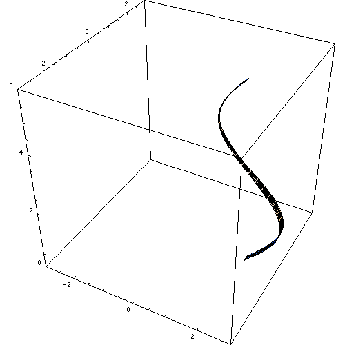Solid of revolutionBy Wikipedia,
the free encyclopedia,

http://en.wikipedia.org/wiki/Solid_of_revolution

In mathematics, engineering, and manufacturing, a solid of revolution is a solid figure obtained by rotating a plane curve around some straight line (the axis) that lies on the same plane.

Assuming that the curve does not cross the axis, the solid's volume is equal to the length of the circle described by the figure's centroid, times the figure's area (Pappus's second centroid Theorem).Rotating a curve

A representative disk is a three-dimensional volume element of a solid of revolution. The element is created by rotating a line segment (of length w) around some axis (located r units away), so that a cylindrical volume of πrw units is enclosed.

## Methods of finding the volume

Two common methods for finding the volume of a solid of revolution are the disc method and the shell method of integration. To apply these methods, it is easiest to draw the graph(s) in question, identify the area that is actually being revolved about the axis of revolution, and then draw a straight line, vertical (parallel to the y-axis) for functions defined in terms of x and horizontal (parallel to the x-axis) for functions defined in terms of x, which is referred to as a slice. Although all formulas are listed in terms of x, the formulas are exactly the same for functions defined in terms of y (with rotations about the x- and y-axes appropriately swapped).

### Disc method

The disc method is used when the slice that was drawn is perpendicular to the axis of revolution; i.e. when integrating along the axis of revolution.

The volume of the solid formed by rotating the area between the curves of f(x) and g(x) and the lines x = a and x = b about the x-axis is given by$V = \pi \int_a^b \vert f(x)^2 - g(x)^2\vert\,dx$

If g(x) = 0 (e.g. revolving an area between curve and x-axis), this reduces to:$V = \pi \int_a^b f(x)^2\,dx$

The method can be visualized by considering a thin vertical rectangle at x between y = f(x) on top and y = g(x) on the bottom, and revolving it about the x-axis; it forms a ring (or disc in the case that g(x) = 0), with outer radius f(x) and inner radius g(x). The area of a ring is π(Rr), where R is the outer radius (in this case f(x)), and r is the inner radius (in this case g(x)). Summing up all of the areas along the interval gives the total volume.

### Cylinder method

The cylinder method is used when the slice that was drawn is parallel to the axis of revolution; i.e. when integrating perpendicular to the axis of revolution.

The volume of the solid formed by rotating the area between the curves of f(x) and g(x) and the lines x = a and x = b about the y-axis is given by$V = 2\pi \int_a^b x\vert f(x) - g(x)\vert\,dx$

If g(x) = 0 (e.g. revolving an area between curve and y-axis), this reduces to:$V = 2\pi \int_a^b x f(x)\,dx$

The method can be visualized by considering a thin vertical rectangle at x with height [f(x) − g(x)], and revolving it about the y-axis; it forms a cylindrical shell. The lateral surface area of a cylinder is rh, where r is the radius (in this case x), and h is the height (in this case [f(x) − g(x)]). Summing up all of the surface areas along the interval gives the total volume.

## External links

Text from Wikipedia is available under the Creative Commons Attribution/Share-Alike License; additional terms may apply.

Published - July 2009

Please see some ads intermixed with other content from this site:Copyright 2004-2021 © by Airports-Worldwide.com, Vyshenskoho st. 36, Lviv 79010, Ukraine
Legal Disclaimer